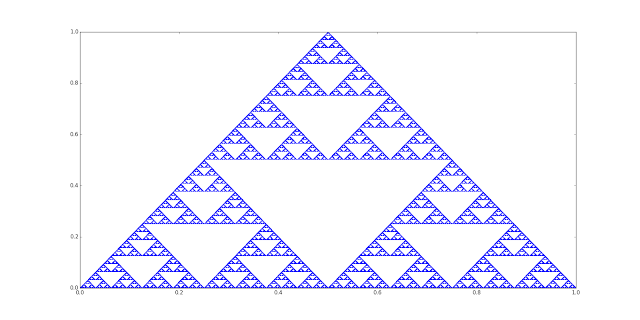## Wednesday, July 22, 2015

### The Chaos Game in polar coordinates: a nice non-Euclidean Sierpinski attractor

I am learning the basic concepts about the Chaos Game (I did a previous question about the same topic at MSE here), the method to create fractals elaborated by professor Michael Barnsley.

The basic example (euclidean-cartesian) requires three attractor vertices, ABC, and as Wikipedia explains: "The fractal is created by iteratively creating a sequence of points, starting with the initial random point, in which each point in the sequence is a given fraction of the distance between the previous point and one of the vertices of the polygon; the vertex is chosen at random in each iteration."

The sequence of points (x,y) will produce the pattern of the Sierpinski attractor:When I saw that construction, I wondered if there was another way of obtaining an attractor with two variables and three attractor points, and thought about polar coordinates. I did not find any references, so I did my own version of the Chaos Game for polar coordinates.

In this version, the points are (theta,r) (the angle in radians and the radius). And the three attractor points are A=(0,0), B=(5PI/4,1) and C=(7PI/4,10^4).

This is the algorithm I have implemented:

And this is the result for 10^7 iterations, a nice Sierpinski attractor:

The Sierpinski attractor appears, but there is a distortion due to the use of polar coordinates. In the case of the polar coordinates the point (theta, r) has two options to arrive to the attractor point: using the clockwise angle or the counterclockwise angle. Only selecting the shortest path (in this case the smallest angle of both options) the Sierpinski attractor is shown. It looked like a (sorry if I am not using the appropriated words) "non-Euclidean" Sierpinski attractor.

This is another example locating the attractor points in the same axis: A=(0,0), B=(PI-PI/8,1) and C=(7PI/4,10^4).

I wondered if, according to the results, can be said that the Sierpinski attractor in the polar coordinates pattern is a "non-Euclidean" version of the attractor (or it is only a "Euclidean" distortion of the original attractor). Fortunately a fellow from MSE confirmed that it is fair to say that is is a non-Euclidean version! Here is the link to my question and the answer. I am very happy because I think this might be the first version of the Chaos Game in polar coordinates showing the Sierpinski attractor in a non-Euclidean "flavor", I could be wrong but initially I did not find it in Internet!